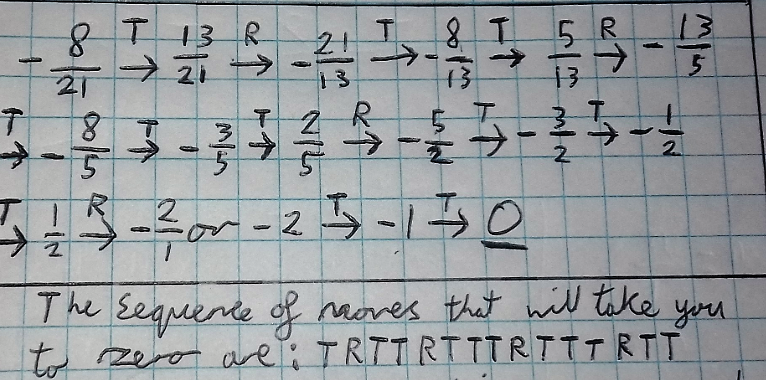#### You may also like### Tweedle Dum and Tweedle Dee

Two brothers were left some money, amounting to an exact number of pounds, to divide between them. DEE undertook the division. "But your heap is larger than mine!" cried DUM...### Sum Equals Product

The sum of the numbers 4 and 1 [1/3] is the same as the product of 4 and 1 [1/3]; that is to say 4 + 1 [1/3] = 4 ï¿½ 1 [1/3]. What other numbers have the sum equal to the product and can this be so for any whole numbers?### Special Sums and Products

Find some examples of pairs of numbers such that their sum is a factor of their product. eg. 4 + 12 = 16 and 4 × 12 = 48 and 16 is a factor of 48.

# Twisting and Turning

##### Age 11 to 14Challenge Level

Ben from the UK, Arkadiusz from the Costello School in the UK and Ved from WBGS in the UK worked out how the rope ends up after the series of moves
Twist, twist, twist, turn, twist, twist, twist, turn, twist, twist, twist, turn
Ben wrote:
Having explored the use of twisting and turning I used the example given to
help me solve the first problem. I found an answer of $-\frac8{21}$ having gone through the sequence of
$1,2,3,-\frac13,\frac23,1 \frac23,2 \frac23,-\frac38,\frac58,1 \frac58,2 \frac58$ to finally get to $-\frac8{21}$

Arkadiusz and Ved also worked out how to get back to $0$. This is Arkadiusz' work:Mohit from Burnt Mill Academy, Harlow in the UK untangled ropes starting from a different state:
TANGLE :
$-\frac{11}{30}$
UNTANGLE:
$-\frac{11}{30}+1=\frac{19}{30}, \\ \frac{19}{30} \rightarrow -\frac1x=-\frac{30}{19},\\ -\frac{30}{19}+1=-\frac{11}{19},\\ -\frac{11}{19}+1=\frac8{19},\\ \frac8{19}\rightarrow -\frac1x=-\frac{19}8, \\ -\frac{19}8+1=-\frac{11}8, \\ -\frac{11}8+1=-\frac38, \\ -\frac38+1=\frac58,\\ \frac58\rightarrow-\frac1x= -\frac85,\\ -\frac85+1=-\frac35, \\ -\frac35+1=\frac25, \frac25\rightarrow-\frac1x=-\frac52, \\ -\frac52+1=-\frac32,\\ -\frac32+1=-\frac12,\\ -\frac12+1=\frac12, \\ \frac12\rightarrow-\frac1x=-2, \\ -2+1=-1, \\ -1=1=0$
T,R,T,T,R,T,T,T,R,T,T,R,T,T,T,R (T,T)

Arkadiusz described a method to untangle ropes starting from any position:

We keep twisting until we get a positive value, then we turn and repeat over and over until we get zero.